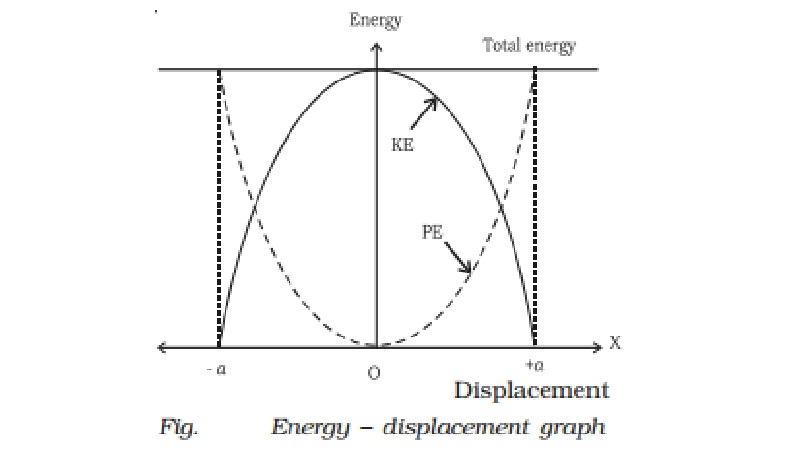Home | | Physics | Kinetic and Potential in simple harmonic motion

# Kinetic and Potential in simple harmonic motionThe total energy (E) of an oscillating particle is equal to the sum of its kinetic energy and potential energy if conservative force acts on it. The velocity of a particle executing SHM at a position where its displacement is y from its mean position is v = ω rt( a2-y2)

Energy in simple harmonic motion

The total energy (E) of an oscillating particle is equal to the sum of its kinetic energy and potential energy if conservative force acts on it. The velocity of a particle executing SHM at a position where its displacement is y from its mean position is v = ω rt( a2-y2)

Kinetic energy

Kinetic energy of the particle of mass m is

K = ? n [ω rt( a2-y2) ]

K = ? m [ω2 ( a2-y2) ]     ?.(1)

Potential energy

From definition of SHM F = ?ky the work done by the force during the small displacement dy is dW = −F.dy = −(−ky) dy = ky dy

Total work done for the displacement y is,

W =  ∫ dW = ∫0y ky dy

W =  ∫0y2 y dy

W = 1/ 2 m ω2 y2

This work done is stored in the body as potential energy

U = 1 /2 m ω2 y2

Total energy E = K + U

= 1/ 2 mω2 (a2 − y2) + 1/ 2 m ω2 y2

= 1/ 2 m ω2 a2

Thus we find that the total energy of a particle executing simple harmonic motion is 1 /2 m ω2 a2

Special cases

(i)When the particle is at the mean position y = 0, from eqn (1) it is known that kinetic energy is maximum and from eqn. (2) it is known that potential energy is zero. Hence the total energy is wholly kinetic

E=Kmax = 1/ 2 mω2a2

(ii) When the particle is at the extreme position y = +a, from eqn. (1) it is known that kinetic energy is zero and from eqn. (2) it is known that Potential energy is maximum. Hence the total energy is wholly potential.

E = Umax = ? m ω2 a2

(iii)when y = a/2  ,

K = 1 /2 m ω2 [a2  -  a2/4]

K = ?(1/2mω2 a 2)

K = 3 /4 E

U = 1/2 mω2 (a 2/2) = ?(1/2 mω2 a 2)

U = 1/4E

If the displacement is half of the amplitude, K = 3/ 4 E and U = 1 /4 E. K and U are in the ratio 3 : 1,

E=K+U=1/2 mω2 a 2

At any other position the energy is partly kinetic and partly potential.

This shows that the particle executing SHM obeys the law of conservation of energy.

Graphical representation of energy

The values of K and U in terms of E for different values of y are given in the Table 6.2. The variation of energy of an oscillating particle with the displacement can be represented in a graph as shown in the Fig..Energy of SHM

Y : 0  a/2 , a , -a/2 , -a

Kinetic energy : E , ? E , 0  ? E, 0

Potential energy : 0 , 1/4E,  E , 1/4E , E

Study Material, Lecturing Notes, Assignment, Reference, Wiki description explanation, brief detail
11th 12th std standard Class Physics sciense Higher secondary school College Notes : Kinetic and Potential in simple harmonic motion |